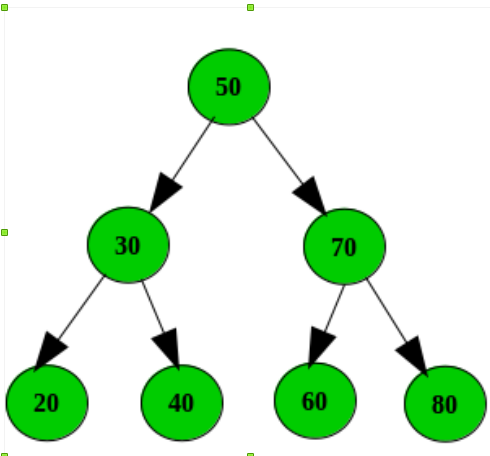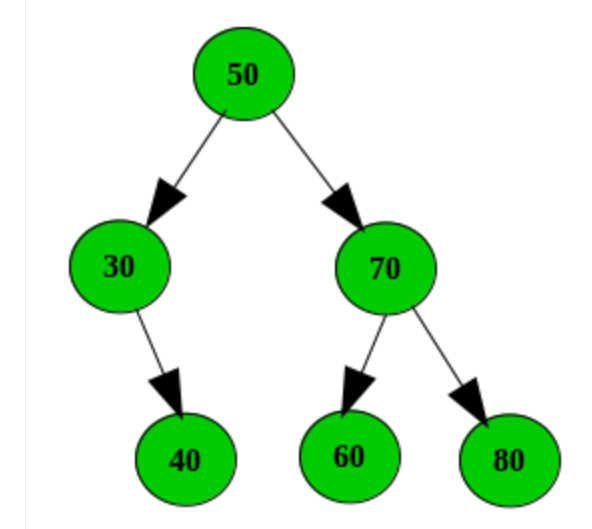# Amazon Interview Experience

Round: I
An Array arr={7, 7, 8, 8, 9, 1, 1, 4, 2, 2} has numbers appearing twice or once. Duplicates appear side by side everytime. Might be few numbers can be occur one time and just assume this is a right rotating array (just say an array can rotate k times towards right). Aim is to identify numbers that occured once in array.

 `#include ` ` `  `int` `main() ` `{ ` `    ``int` `a[] = { 7, 7, 8, 8, 9, 1, 1, 4, 2, 2 }, i = 1, m = 10; ` `    ``// int a[]={7, 8, 8, 9, 1, 1, 4, 2, 2, 7}, i=1, m=10; ` ` `  `    ``if` `(a == a[m - 1]) { ` `        ``i = 2; ` `        ``m = m - 1; ` `    ``} ` `    ``else` `        ``printf``(``"%d\n"``, a[m - 1]); ``// For cases like { 7, 7, 8, 8, 9, 1, 1, 4, 2, 3 }, a[] = { 7, 7, 8, 8, 9, 1, 1, 4, 4, 2 } ` `    ``for` `(; i < m; i++) ` `        ``if` `(a[i] == a[i - 1]) ` `            ``i++; ` `        ``else` `            ``printf``(``"%d\n"``, a[i - 1]); ` ` `  `    ``return` `0; ` `} `

Input:
7, 7, 8, 8, 9, 1, 1, 4, 2, 2
Output:
9
4

Round II:
In second round a question asked was asked regarding BST. Sum of key elements in individual path of BST (just say this sum as path_ weight) and threshold path weight is given as input. If any of the pathweight is less than oversold path weight then that should be deleted from the tree.

Example :
Input:
Consider the below tree as input and threshold path weight is 110.Output:
Below are the no of paths the input tree can make
path 1 : 50 -> 30 -> 20, Sum = 100
path 1 : 50 -> 30 -> 40, Sum = 120
path 1 : 50 -> 70 -> 60, Sum = 180
path 1 : 50 -> 70 -> 80, Sum = 200

In the current scenario path is less than the threshold path weight (100 < 110), So we have to destroy the path 1.
Tree after destroying the path 1.`#include ` `#include ` ` `  `struct` `node { ` `    ``int` `key; ` `    ``struct` `node *left, *right; ` `}; ` ` `  `struct` `node* newnode(``int` `element) ` `{ ` `    ``struct` `node* temp = (``struct` `node*)``malloc``(``sizeof``(``struct` `node)); ` `    ``temp->key = element; ` `    ``temp->left = temp->right = NULL; ` `    ``return` `temp; ` `} ` ` `  `struct` `node* insert(``struct` `node* root, ``int` `element) ` `{ ` `    ``if` `(root == NULL) ` `        ``return` `newnode(element); ` ` `  `    ``if` `(element < root->key) ` `        ``root->left = insert(root->left, element); ` `    ``else` `if` `(element > root->key) ` `        ``root->right = insert(root->right, element); ` ` `  `    ``return` `root; ` `} ` ` `  `void` `inorder(``struct` `node* mynode) ` `{ ` `    ``if` `(mynode != NULL) { ` `        ``inorder(mynode->left); ` `        ``printf``(``"%d\t"``, mynode->key); ` `        ``inorder(mynode->right); ` `    ``} ` `} ` ` `  `struct` `node* minValueNode(``struct` `node* node) ` `{ ` `    ``struct` `node* current = node; ` ` `  `    ``/* loop down to find the leftmost leaf */` `    ``while` `(current->left != NULL) ` `        ``current = current->left; ` ` `  `    ``return` `current; ` `} ` ` `  `struct` `node* deleteNode(``struct` `node* root, ``int` `key) ` `{ ` `    ``// base case ` `    ``if` `(root == NULL) ` `        ``return` `root; ` ` `  `    ``// If the key to be deleted is smaller than the root's key, ` `    ``// then it lies in left subtree ` `    ``if` `(key < root->key) ` `        ``root->left = deleteNode(root->left, key); ` ` `  `    ``// If the key to be deleted is greater than the root's key, ` `    ``// then it lies in right subtree ` `    ``else` `if` `(key > root->key) ` `        ``root->right = deleteNode(root->right, key); ` ` `  `    ``// if key is same as root's key, then This is the node ` `    ``// to be deleted ` `    ``else` `{ ` ` `  `        ``// node with only one child or no child ` `        ``if` `(root->left == NULL) { ` `            ``struct` `node* temp = root->right; ` `            ``free``(root); ` `            ``return` `temp; ` `        ``} ` `        ``else` `if` `(root->right == NULL) { ` `            ``struct` `node* temp = root->left; ` `            ``free``(root); ` `            ``return` `temp; ` `        ``} ` ` `  `        ``// node with two children: Get the inorder successor (smallest ` `        ``// in the right subtree) ` `        ``struct` `node* temp = minValueNode(root->right); ` ` `  `        ``// Copy the inorder successor's content to this node ` `        ``root->key = temp->key; ` ` `  `        ``// Delete the inorder successor ` `        ``root->right = deleteNode(root->right, temp->key); ` `    ``} ` `    ``return` `root; ` `} ` ` `  `/** Function : checkpathCost(int pathweight, int thresoldPathWeight,  ` `                         ``struct node *currentNode, struct node *root) ` `    ``This function will sum the all individual elements in a every  ` `    ``path and lets say it as pathweight and if the sum less than  ` `    ``key then the path will be deleted. ` `     `  `    ``Arguments:  ` `    ``pathweight         : Sum of all elements in a path. As we are ` `                         ``traversing in recursive mode we the sum of  ` `                         ``previews elements. ` `    ``thresoldPathWeight : Threshold value to compare the path weight ` `    ``currentNode        : CUrrent node in the tree. ` `    ``root               : root of the tree, used while deleting the node.   ` ` `  `    ``Output : will delete paths which not satisfy the condition  ` `             ``(pathweightkey; ` ` `  `    ``// Check the conditionh if current node is a leaf node. ` `    ``if` `((currentNode->left == NULL) && (currentNode->right == NULL)) { ` ` `  `        ``// If pathweight is less that thresoldPathWeight delete the path ` `        ``if` `(pathweight < thresoldPathWeight) { ` ` `  `            ``// we need to delete the node, so there is no necessity ` `            ``// in maintaining the current->key in pathweight. ` `            ``pathweight = pathweight - currentNode->key; ` `            ``printf``(``"need to Delete %d.\n"``, currentNode->key); ` `            ``deleteNode(root, currentNode->key); ` `            ``checkpathCost(0, thresoldPathWeight, root, root); ` `        ``} ` `        ``else` ` `  `            ``// If currentNode->key satisfies the condition ` `            ``// (pathweight > thresoldPathWeight), need to backtrack ` `            ``// back to check with other paths and no necessity in ` `            ``// maintaining the current->key in pathweight. ` `            ``pathweight = pathweight - currentNode->key; ` `    ``} ` ` `  `    ``// If currentNode is not a leaf traverse all the ` `    ``// possible paths. ` `    ``if` `(currentNode->left != NULL) { ` `        ``checkpathCost(pathweight, thresoldPathWeight, ` `                      ``currentNode->left, root); ` `    ``} ` `    ``if` `(currentNode->right != NULL) { ` `        ``checkpathCost(pathweight, thresoldPathWeight, ` `                      ``currentNode->right, root); ` `    ``} ` `} ` ` `  `int` `main() ` `{ ` ` `  `    ``int` `thresoldPathWeight = 110; ` ` `  `    ``/* Let us create following BST ` `              ``50 ` `           ``/     \ ` `          ``30      70 ` `         ``/  \    /  \ ` `       ``20   40  60   80 */` ` `  `    ``struct` `node* root = NULL; ` `    ``root = insert(root, 50); ` `    ``root = insert(root, 30); ` `    ``root = insert(root, 20); ` `    ``root = insert(root, 40); ` `    ``root = insert(root, 70); ` `    ``root = insert(root, 60); ` `    ``root = insert(root, 80); ` ` `  `    ``printf``(``"Inorder traversal of the given tree \n"``); ` `    ``inorder(root); ` `    ``printf``(``"\n"``); ` ` `  `    ``checkpathCost(0, thresoldPathWeight, root, root); ` ` `  `    ``printf``(``"Inorder traversal of the given tree \n"``); ` `    ``inorder(root); ` `    ``printf``(``"\n"``); ` ` `  `    ``return` `0; ` `} `

In addition to the existing code for BST in geeksforgeeks and below function will implement the above task.
https://www.geeksforgeeks.org/binary-search-tree-set-2-delete/

Write your Interview Experience or mail it to contribute@geeksforgeeks.orgMy Personal Notes arrow_drop_up

This article is contributed by N.Uday Kiran. If you like GeeksforGeeks and would like to contribute, you can also write an article using contribute.geeksforgeeks.org or mail your article to contribute@geeksforgeeks.org. See your article appearing on the GeeksforGeeks main page and help other Geeks.

Please Improve this article if you find anything incorrect by clicking on the "Improve Article" button below.